# Zeros of derivatives

Much attention focuses on zeros of L-functions, but there are also some interesting questions about zeros of the derivatives of these functions.

In some cases we know more about zeros of the derivatives. For example, Levinson's method [58 #27837][ MR 84g:10070] can be used to show that for any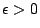there is an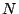such that if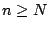then more than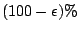the zeros of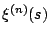are on the critical line, where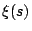is the Riemann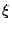-function.

In other cases we know almost nothing. For example, nobody has even made a plausible conjecture about the distribution of the zeros of the derivatives of the Riemann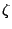-function.

See the articles on derivatives of $\xi(s)$ and derivatives of $\zeta(s)$.

Back to the main index for L-functions and Random Matrix Theory.